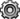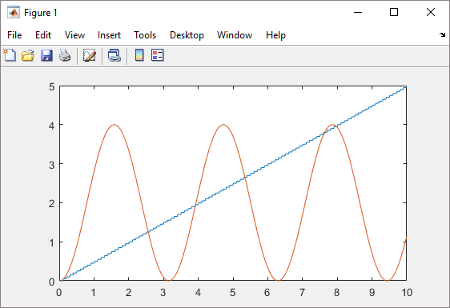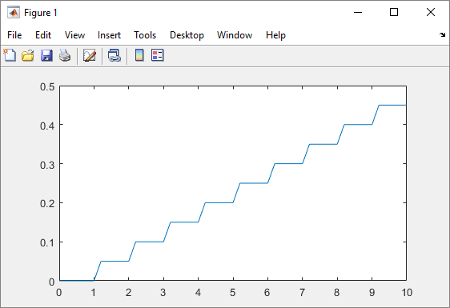Documentation

## Test Export-Function Model Simulation Using Input Matrix

When function-call sequencing is simple enough to be specified as a model input, simulation using an input matrix is the preferred method for testing an export-function model.

• Create time vectors that specify function-call times.

• Create a matrix that adds input data to the time vectors.

• Run simulation.

To create the model in this example, see Create Export-Function Model.

### Create Function-Call Inputs and Data Inputs

Create time-vectors indicating when events occur for root-level function-call Inport blocks and data-vectors for root-level data Inport blocks.

1. For Inport 1 and Inport 2 blocks, create column vectors with time steps of `0.1` and `0.01`. In the MATLAB Command window, enter

```t1 = [0:0.1:10]' t2 = [0:0.01:10]'```
• The time vector data type must be double and monotonically increasing.

• If the sample time for a function-call Inport block is specified, the time vector must be a periodic series increasing with the specified value.

• To specify multiple function-calls at a given time step, repeat the time value accordingly. For example, to specify three events at t = 0.1 and 2 events at t = 0.5, list 0.1 three times and 0.5 twice in your time vector, ```t = [0.1 0.1 0.1 0.5 0.5]'```.

• If you want to use a nonperiodic time vector, set the Inport block Sample time to `-1` and provide a nonperiodic time vector, t1 = [0, 0.1, 0.2, 0.4, 0.8]

2. Create a matrix with time steps and data values for Inport block `3`.

```sine_data = sin(0:0.01:10)' d3 =[t2,sine_data]```

The data input can use any supported format as described in Forms of Input Data.

The following table provides additional information for specifying the time vector `t`.

Sample time type for internal Trigger Port block set to:

Root-level Function-Call Inport block with inherited sample time (-1)

Root-level Function-Call Inport block with discrete sample time

`Triggered`

Nondecreasing column vector.

The function-call subsystem executes at the times specified by the column vector. The sample times can be periodic or aperiodic.

If you specify an empty matrix (`[]`), the function-call subsystem does not execute.

Nondecreasing column vector. Each element in the column vector must be an integer multiple of the sample time specified by the Inport block.

The function-call subsystem executes at the times specified by the column vector.

Alternatively, specify an empty matrix (`[]`) and the function-call subsystem executes at every sample time specified by the Inport block.

`Periodic`

Configuration not allowed.

Empty matrix(`[]`).

The the function-call subsystem executes at the times specified by the Inport block calling it.

### Simulate Export-Function Model

Simulate the export-function model to test and observe its behavior before generating code.

1. Import time and data from the MATLAB® workspace.

On the Modeling tab and from the Setup section, select Model Settings. In the Configuration Parameters dialog box, select the Data Import/Export pane and set the Input parameter to `t1, t2, d3`.

`t1` and `t2` are column vectors containing event times for the function-call Inport blocks `1` and `2`. `d3` is a table of input values versus time for the data Inport block `3`.

2. Run simulation.

3. Plot results. In the MATLAB Command Window, enter.

```plot(yout.time, yout.signals(1).values) hold plot(yout.time, yout.signals(2).values)```4. Change `t1` to provide events every `0.5` seconds. `0.5` is an integer multiple of `0.1` specified in the Inport block 1.

`t1 = [0:0.5:10]'`
5. Rerun simulation.After you test your model, you can generate code for the functions. See Generate Code for Export-Function Model.